## K-Function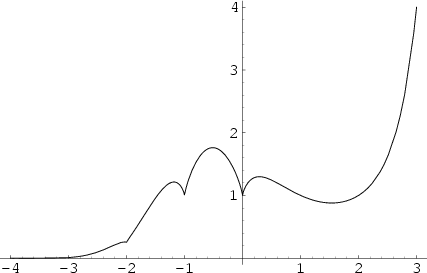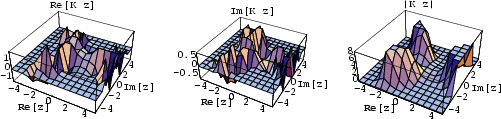An extension of the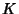-function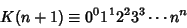(1)

defined by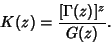(2)

Here,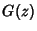is the G-Function defined by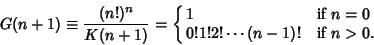(3)

The-function is given by the integral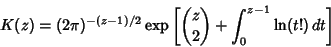(4)

and the closed-form expression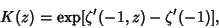(5)

where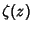is the Riemann Zeta Function,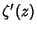its Derivative,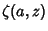is the Hurwitz Zeta Function, and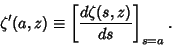(6)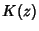also has a Stirling-like series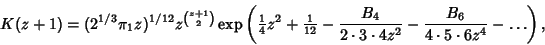(7)

where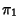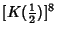(8)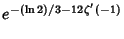(9)(10)

and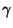is the Euler-Mascheroni Constant (Gosper).

The first few values of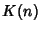for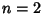, 3, ... are 1, 4, 108, 27648, 86400000, 4031078400000, ... (Sloane's A002109). These numbers are called Hyperfactorials by Sloane and Plouffe (1995).

Sloane, N. J. A. Sequence A002109/M3706 in An On-Line Version of the Encyclopedia of Integer Sequences.'' http://www.research.att.com/~njas/sequences/eisonline.html and Sloane, N. J. A. and Plouffe, S. The Encyclopedia of Integer Sequences. San Diego: Academic Press, 1995.